# Long Division with Remainders Worksheets 4th Grade

📆 23 May 2022
📂 Gallery Type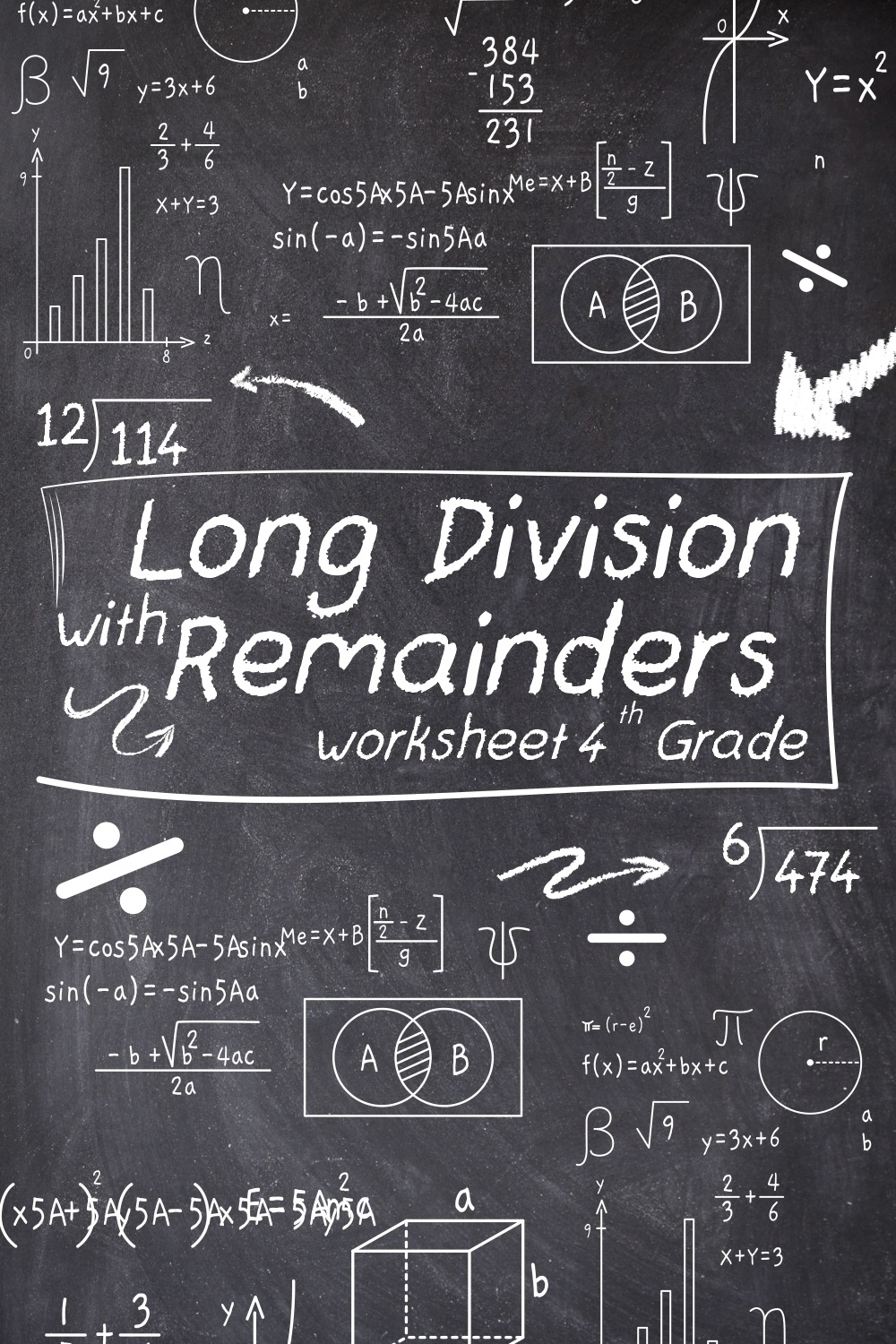12 Images of Long Division With Remainders Worksheets 4th Grade

Long Division with Remainders Worksheets 4th Grade here! Use our worksheet to teach your student!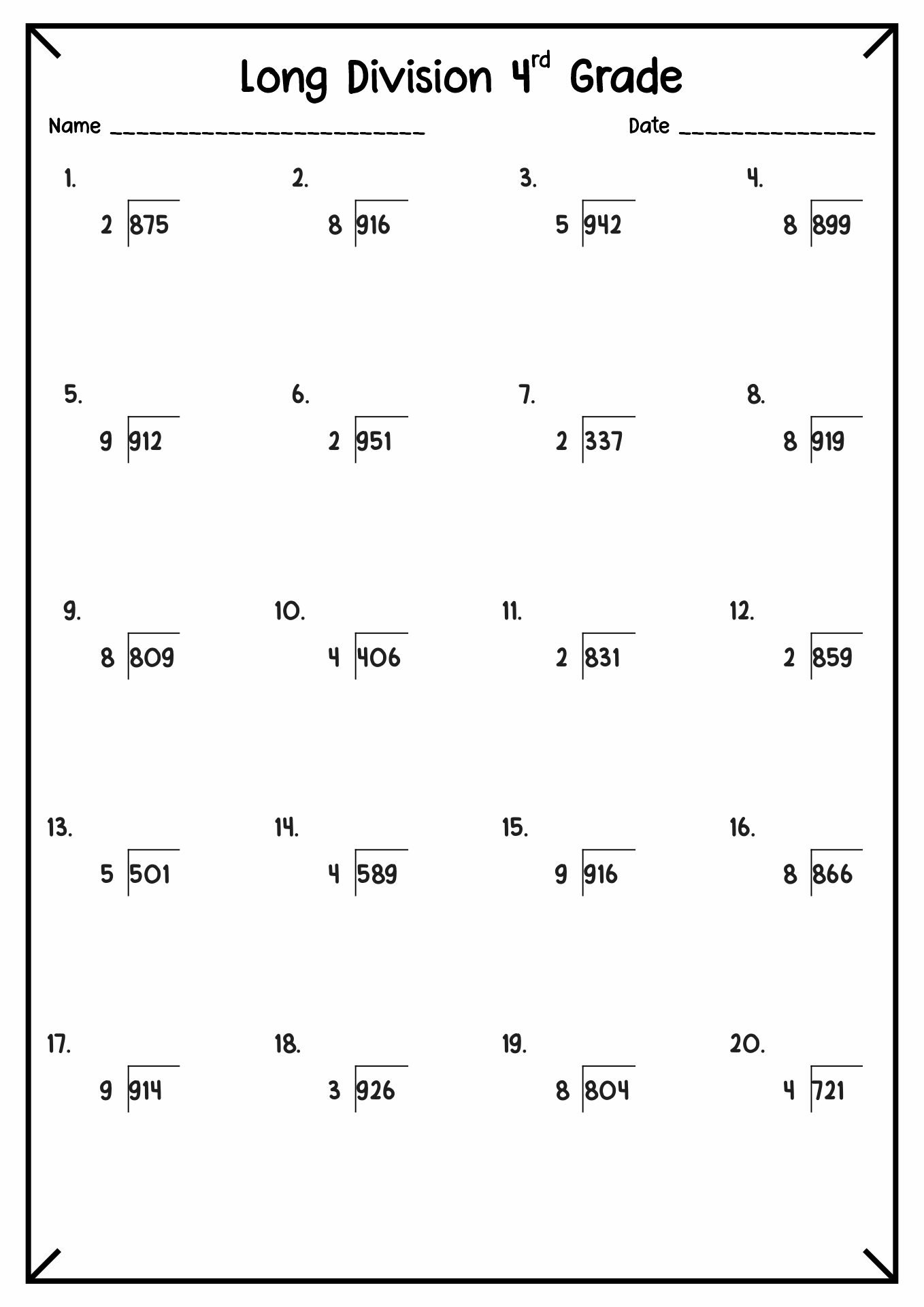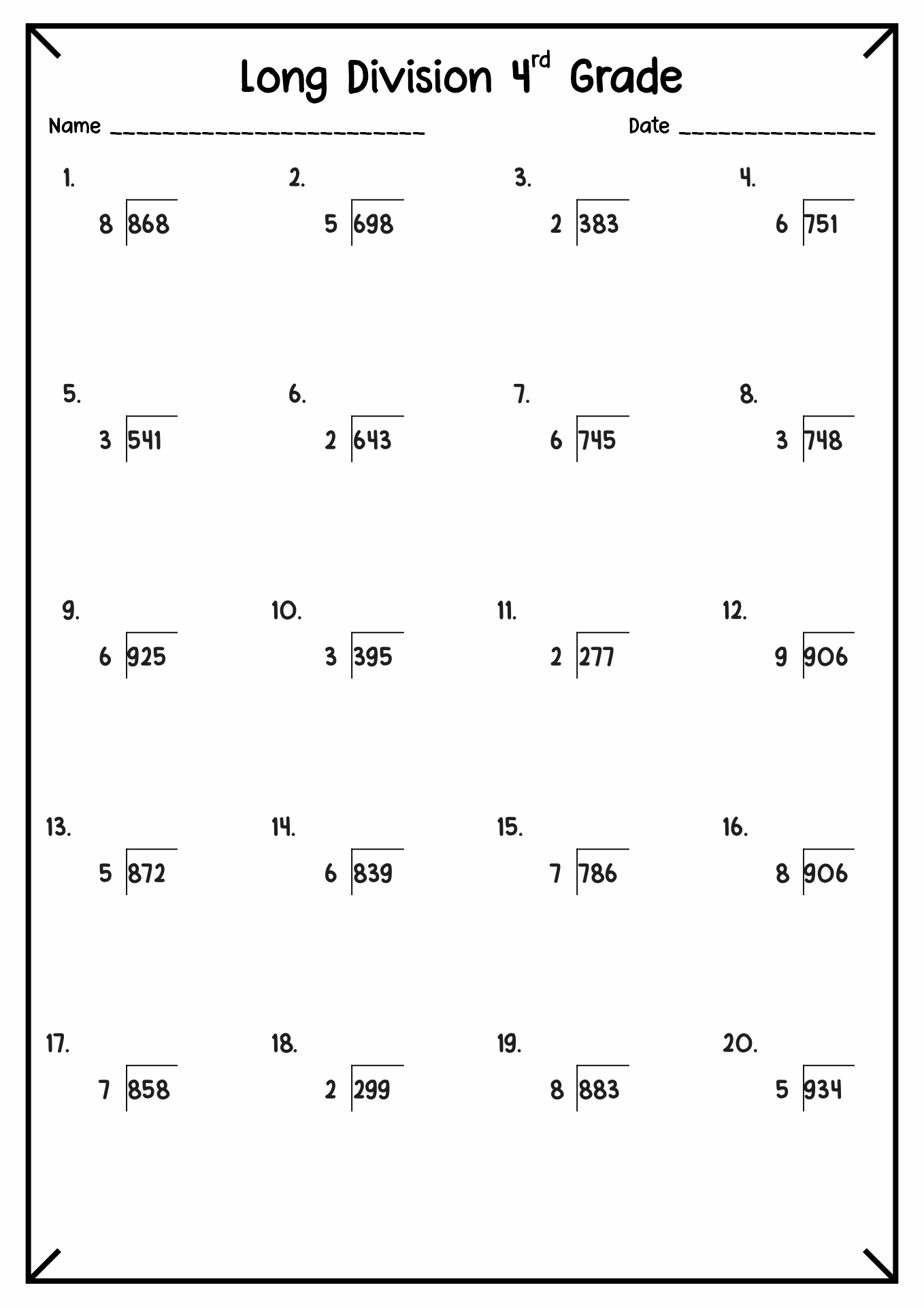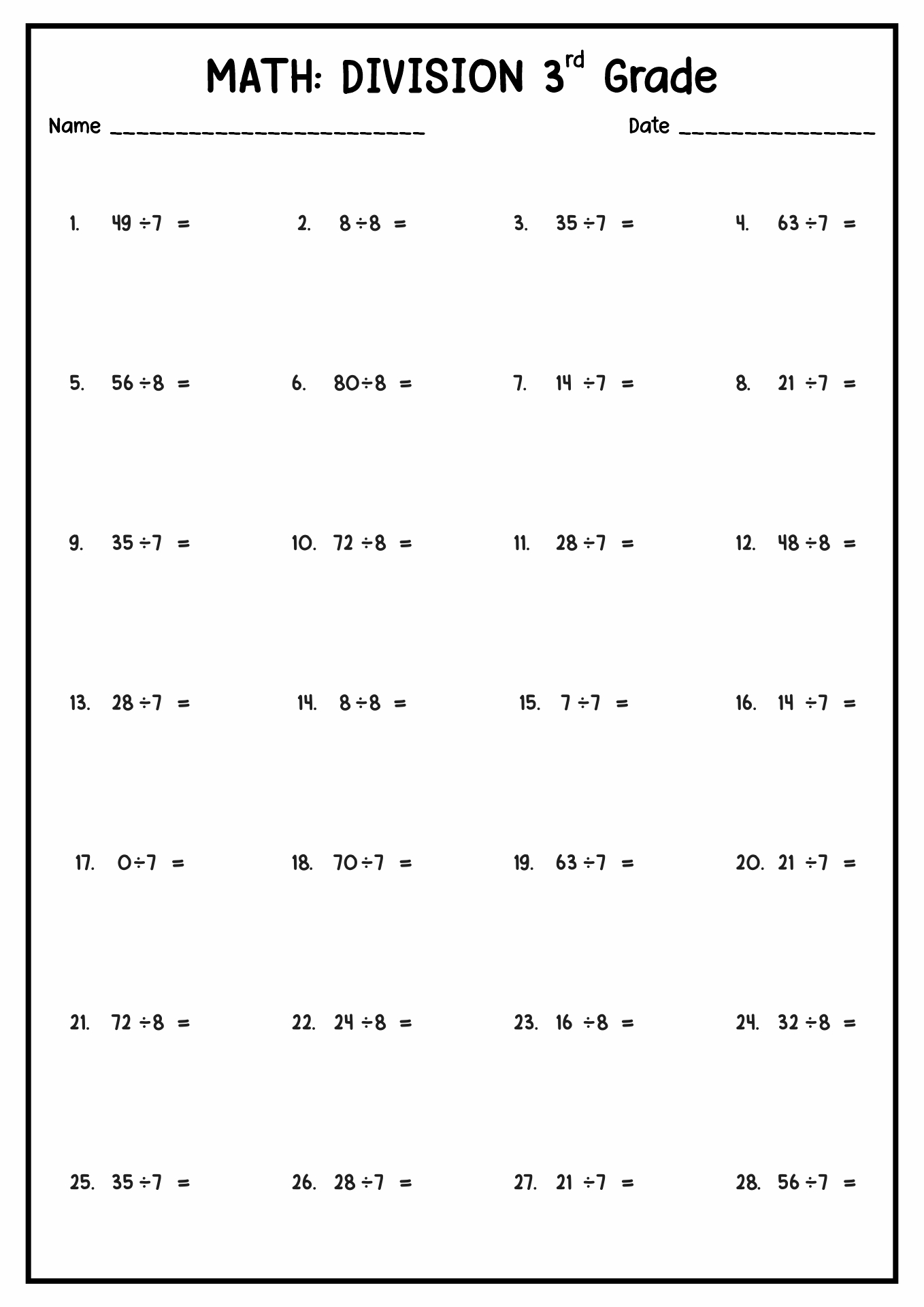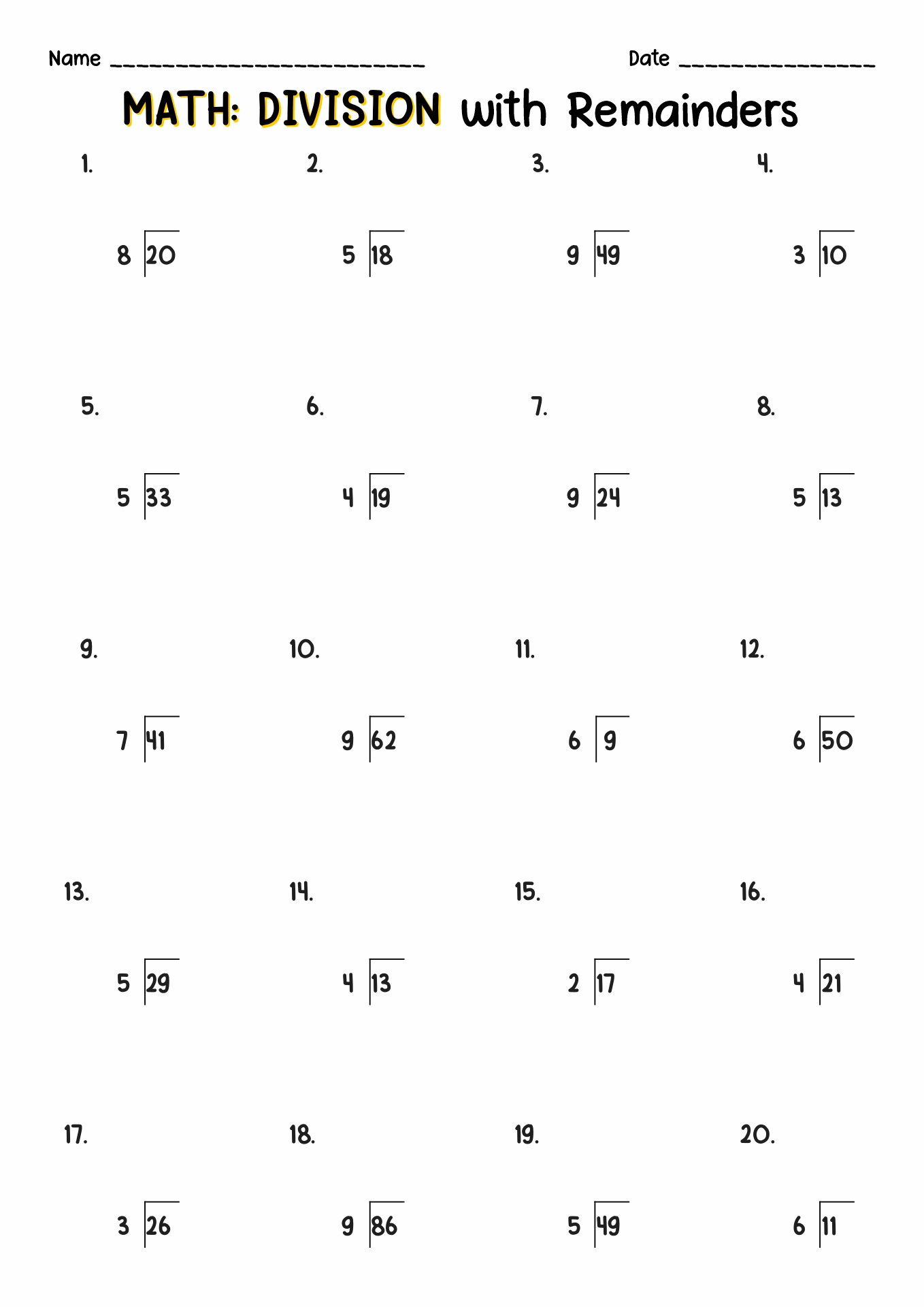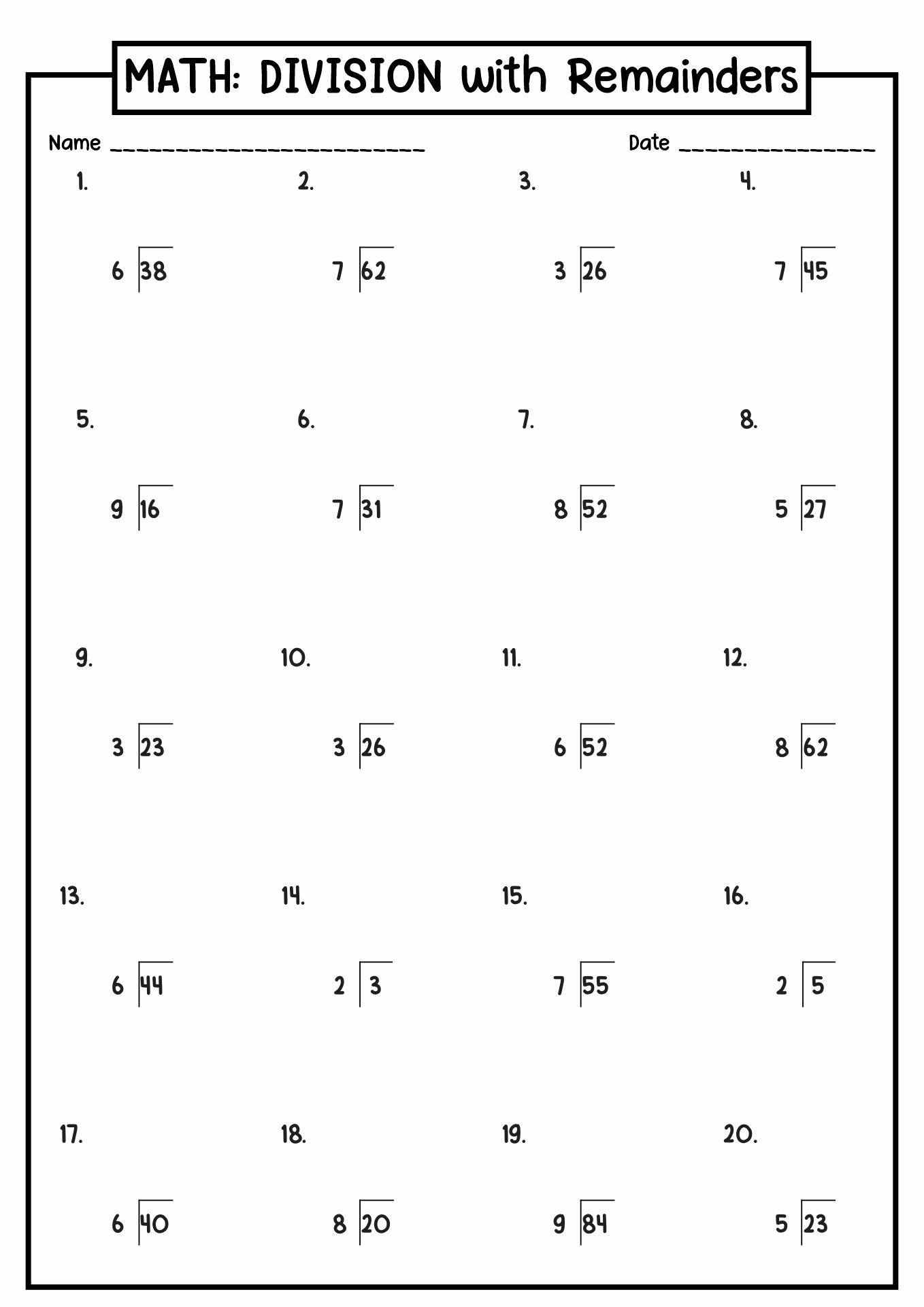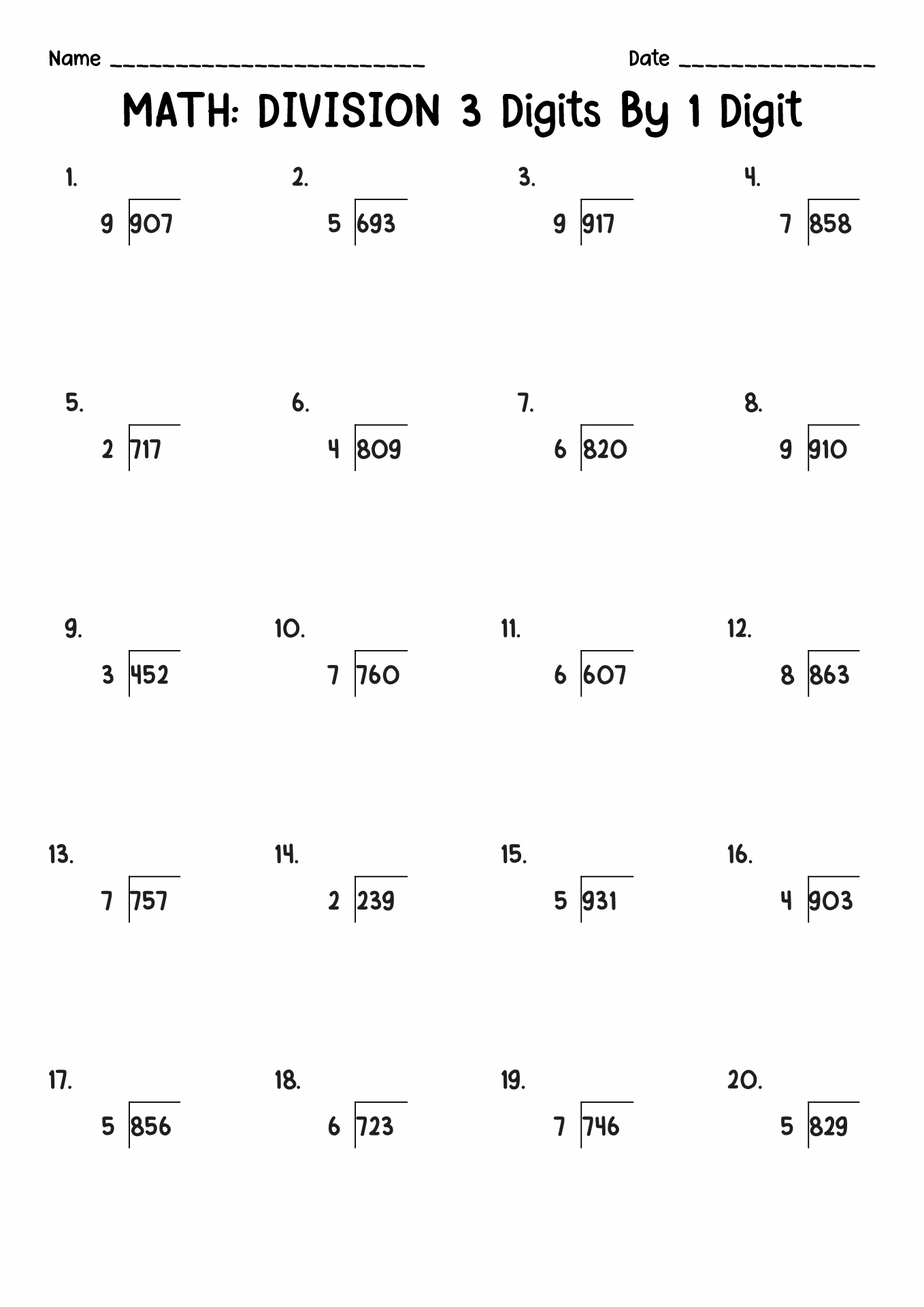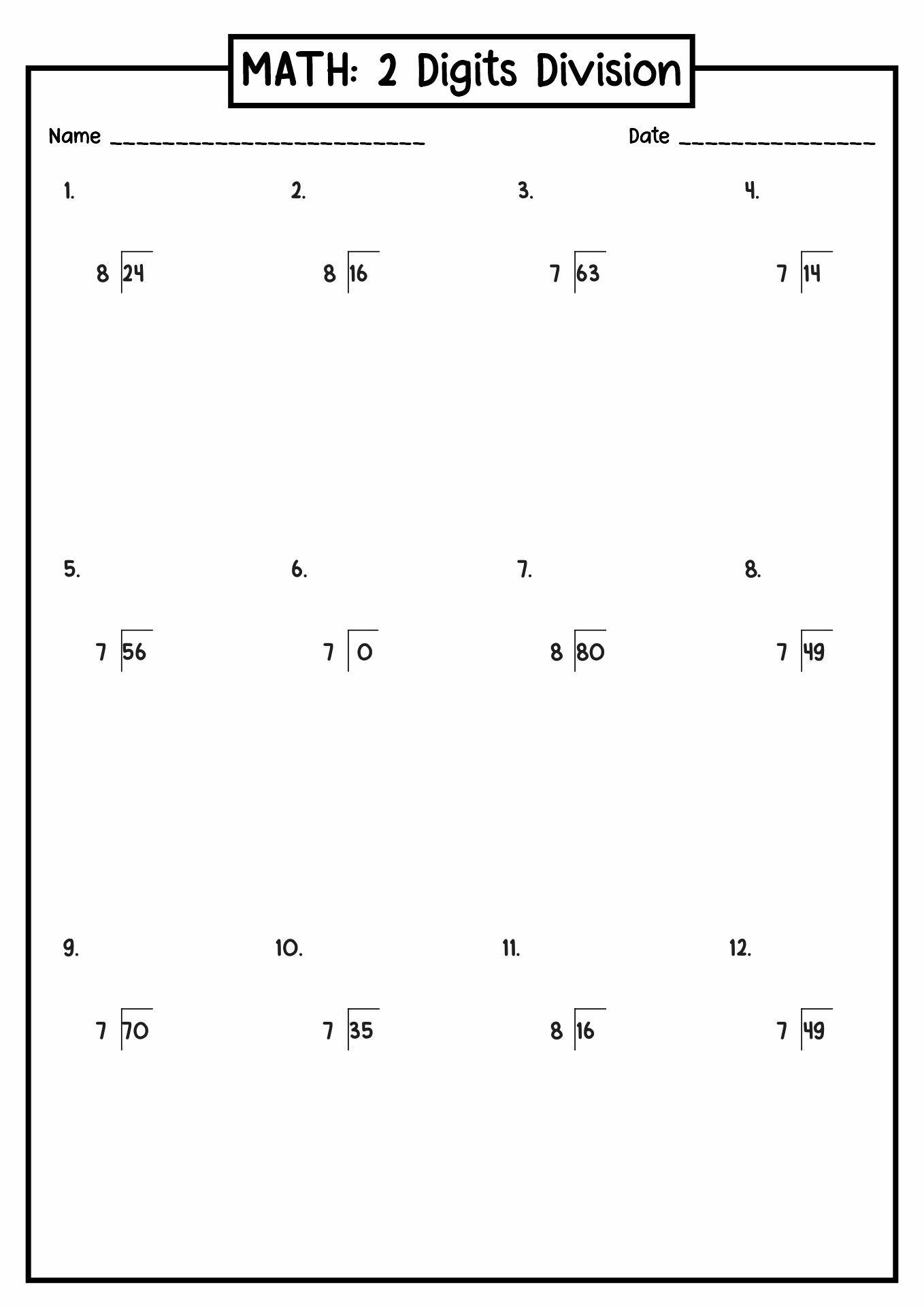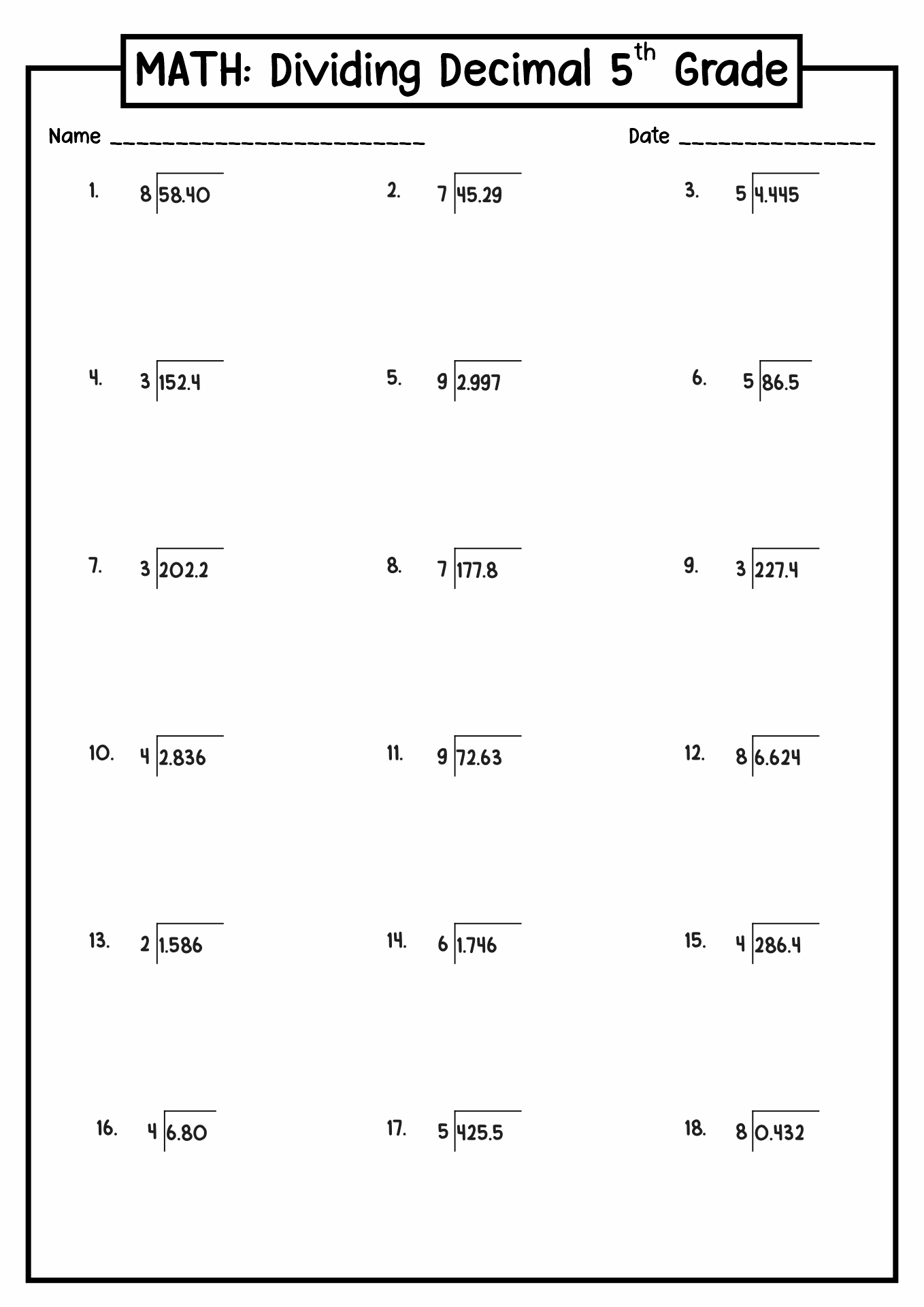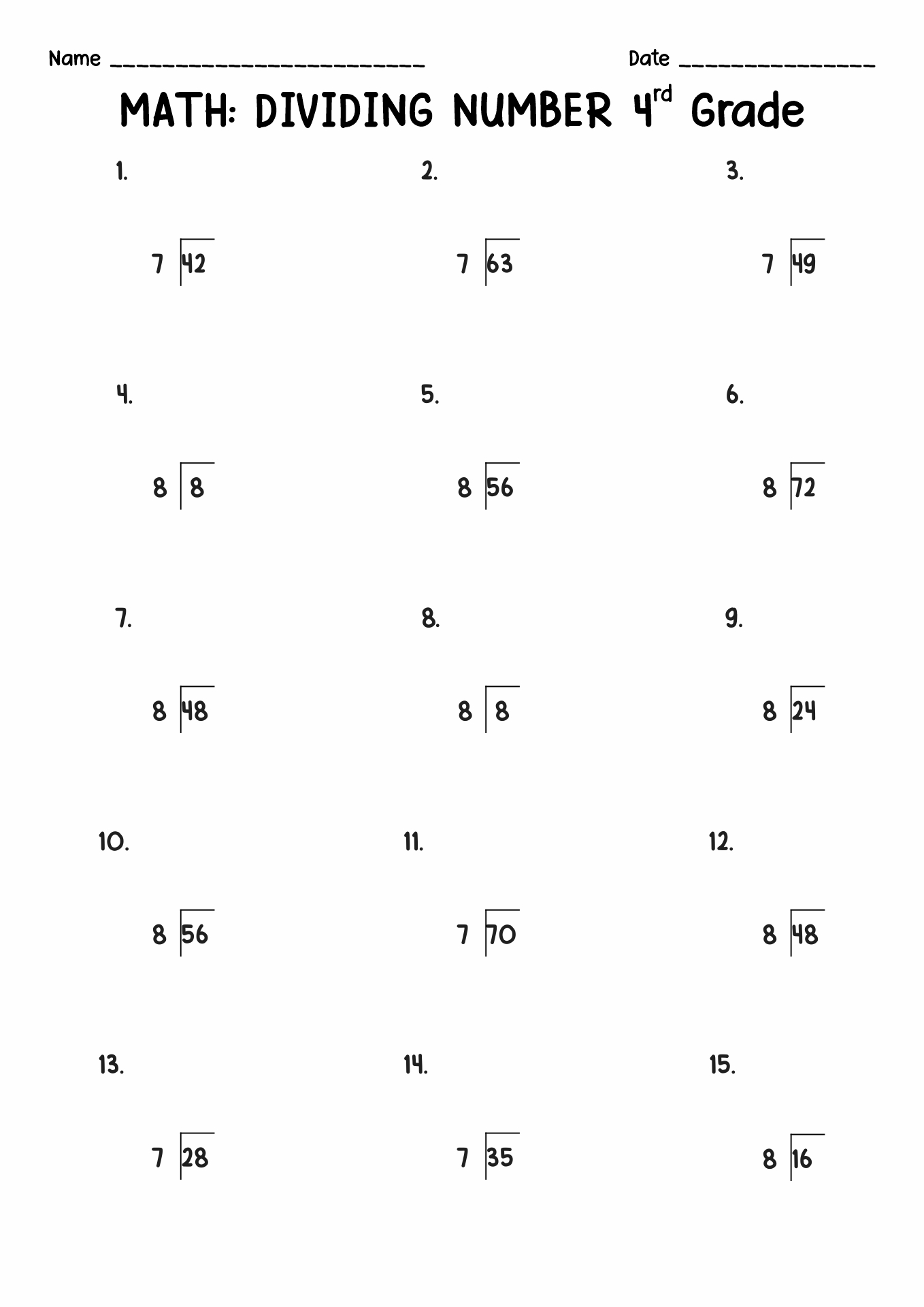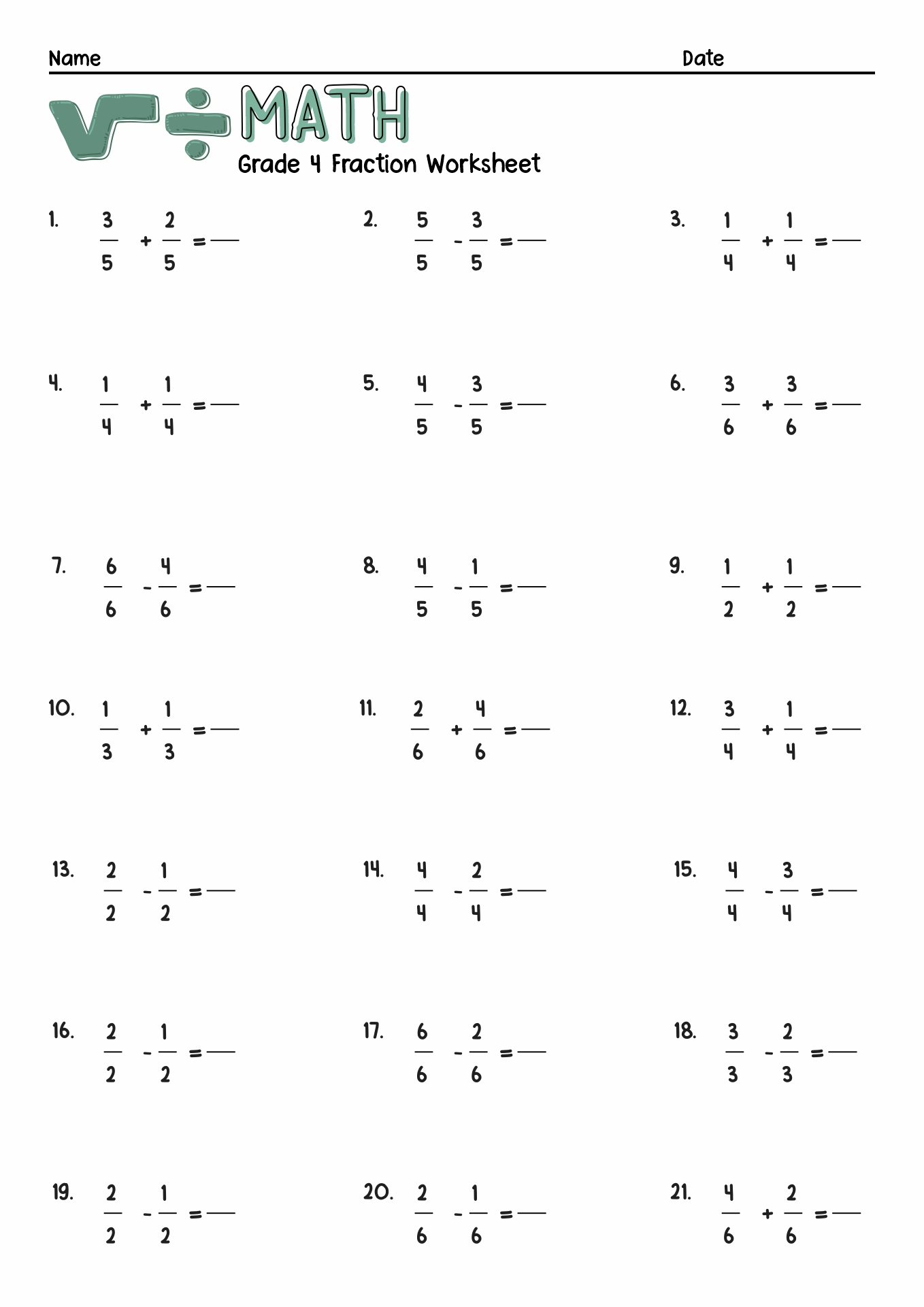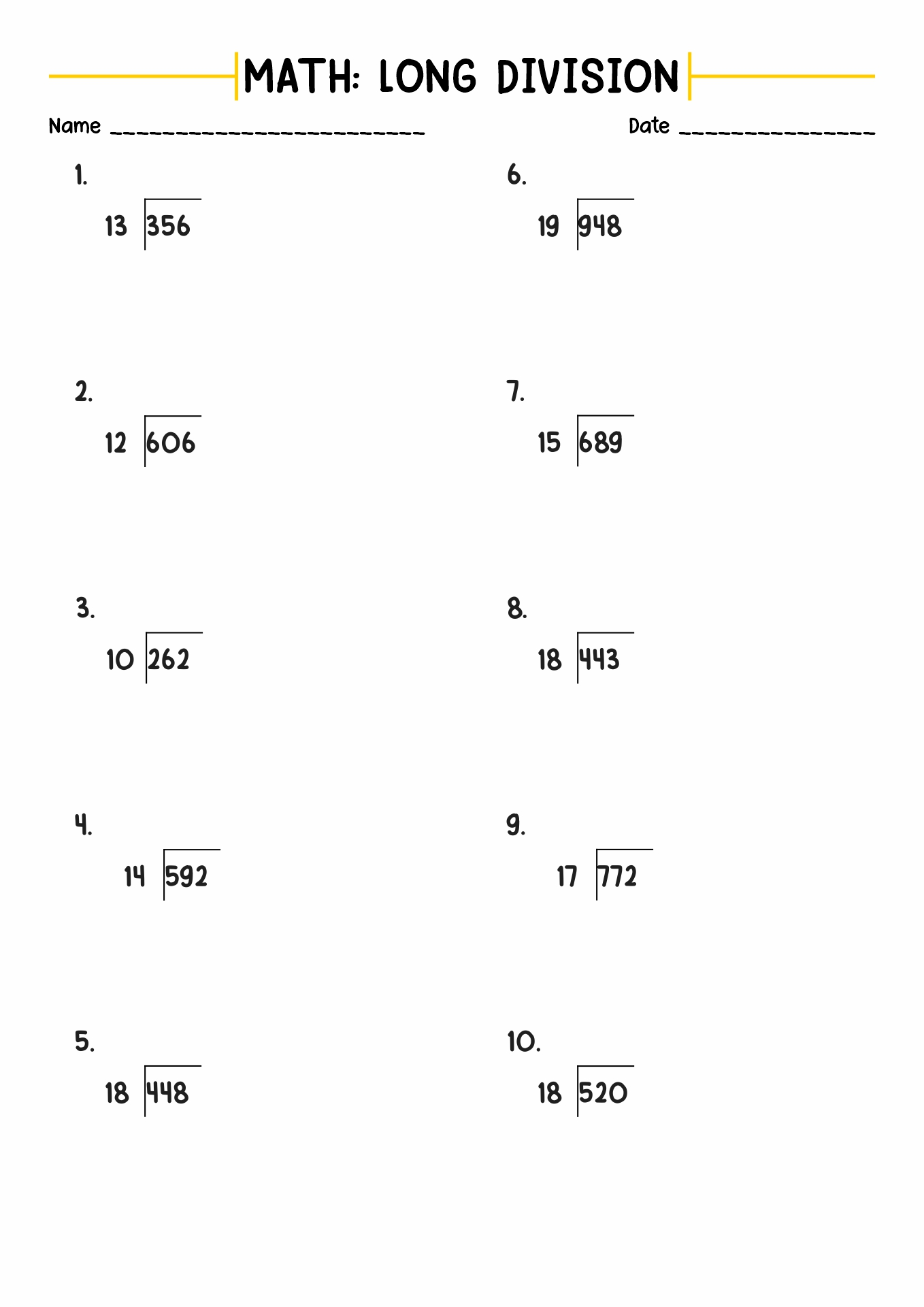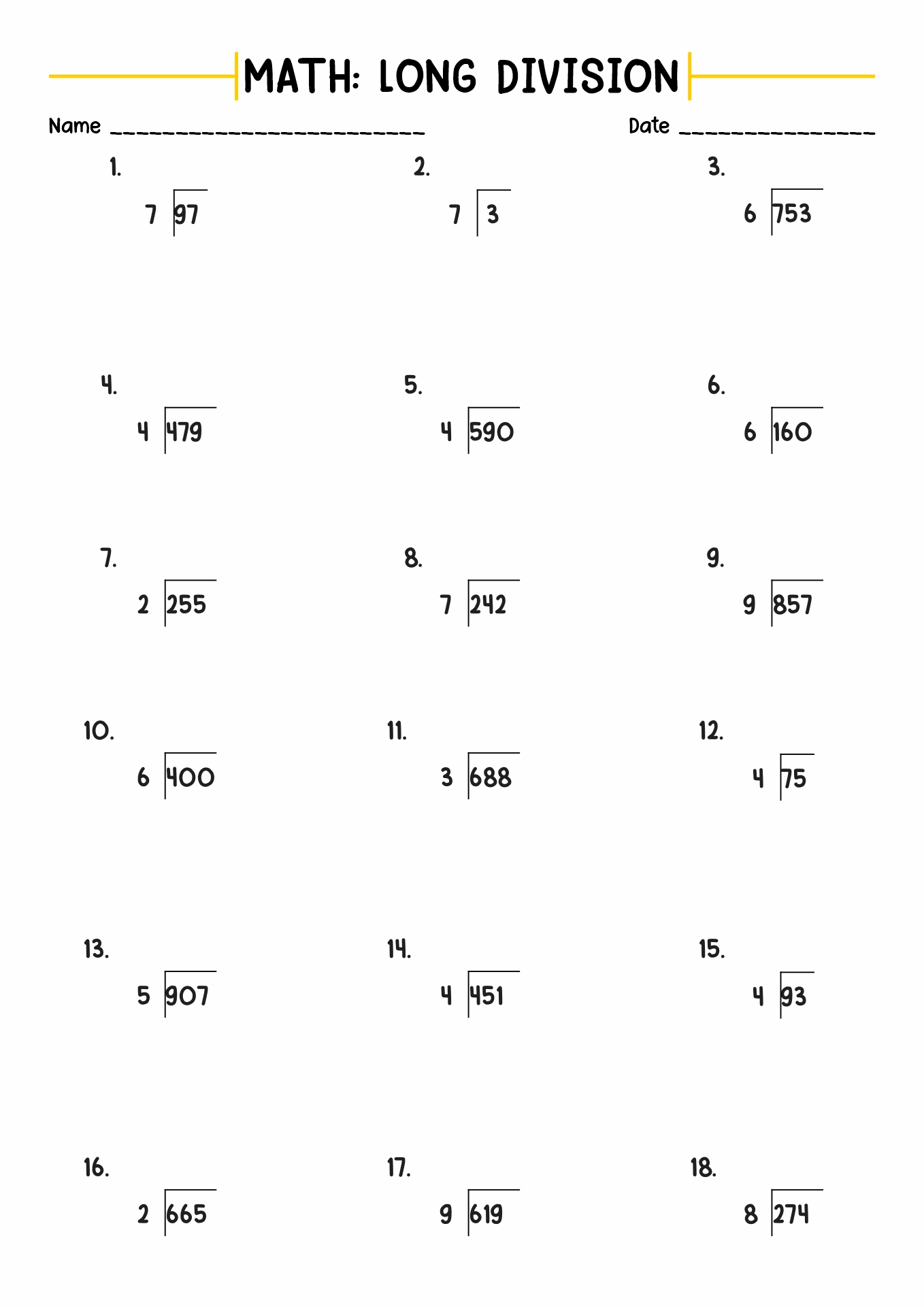Solve the complex division problem with these Long Division with Remainders Worksheets 4th Grade!

Summary: In the mathematical sense, a division is a method of breaking a number into two similar amounts. There are two strategies to solve the division, short and long division. It is a method to solve a complicated division with two or more numbers. When solving division, sometimes we cannot divide all the numbers perfectly. There is an excess value of the division process called the remainder. Learning long division with remainders will help students to solve the complex mathematical question and prepare them for future education.

### What is the Introduction of Division?

In mathematics, there are four foundation formulas to solve every numerical problem, known as arithmetic operations. There are four basic arithmetic operations, addition, subtraction, multiplication and division. Young students will learn all these operations in elementary school. Even though there are four operations, they have various variations for each. Mastering the basic arithmetic operations will help young learners with more advanced mathematical problems in later education.

In this article, we will talk about division. In the mathematical sense, a division is a method of breaking a number into two similar amounts. The symbol of this formula consists of a horizontal line with two dots above and below it (÷) or a slash (/). There are two popular types of a division operations, partitive and quotative. The division has three parts or elements (dividend, divisor and quotient). There are two strategies to solve the division, short and long division.

### What is the Definition of Long Division?

Long division is one of the strategies for solving mathematical division problems. Many mathematicians recommend using this method to solve a complicated division with two or more numbers. It also can be used to work on the question with decimal numbers and to calculate a remainder of a division. According to David Klein from California State University, learning long division help students to build conceptual grounds for mathematics. The long division has three elements which help students understand the context (dividend, divisor, and quotient). A dividend is a number to be divided, dividends are the number which divides the dividend, and the quotient is the final result of the problem. The long division also has another element called the remainder. The long division does not use the slash (/) or division sign (÷). Instead, it uses the right parenthesis < ) > and a horizontal line. The mathematicians refer to these signs as a long division symbol or a division bracket.

### What are the Types of Long Division?

In mathematics, long division is a method for dividing large numbers into smaller groups. It simplifies complex mathematical problems into several easy steps. Understanding the context of long division will help students to develop their numerical skills and brain capacity. In Long division, there are some methods to solve mathematical problems, digit-based method, chunking method, bidirectional chunking method, freeform method, negative divisor problem and scaling division.

### What are Remainders?

When solving division, sometimes we cannot divide all the numbers perfectly. There is an excess value of the division process called the remainder. For example, when we divide ten (10) by four (4), we can only divide it into two parts, and there is an excess number (2). We can write the problem as 10: 4 = 2 R2. The letter "R" is the symbol of the remainder. To use it, write the capital "r" and put the remainder number after it. Based on the statement by Sebastian Pauli from the University of North Carolina, the remainder is the leftover number from the division of a number by b number. The rule method for this problem is "dividend - (divisor x quotients) = remainder". There are various techniques for writing the remainder. Using whole numbers or a fraction are two examples of the method. Most people use the whole number method; however, you can use whatever you want. Writing the remainder as a fraction is relatively simple. Put the leftover number as a numerator and the divisor as the denominator. The part of the result and the excess number is dividend and divisor (dividend-per-divisor).

### How to Solve a Long Division with Remainders Problem?

In learning long division, some students struggle because of the complexity of the problem. It is one of the challenges that teachers face in the classroom. Plus, some students already felt demotivated when they entered the math class. To overcome this problem, math teachers should be creative in teaching. Below are the steps for solving the long-division question

1. Arrange the long division set-up by writing the divisor outside the dividing box and the dividend inside.
2. Start to split the dividend by the divisor.
3. Multiply the incomplete result number with the divisor.
4. Examine and analyze the differences and ensure it should be in a smaller number than the divisor.
5. Pull down the next number on the dividend and repeat the first step.

The information, names, images and video detail mentioned are the property of their respective owners & source.

### Popular Categories

Have something to tell us about the gallery?

Submit